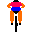# Prof. Dr. Gabriele Nebe

Office: Pontdriesch 14/16, room 117
E-mail: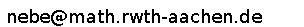Lehrstuhl D für Mathematik
RWTH Aachen
52056 Aachen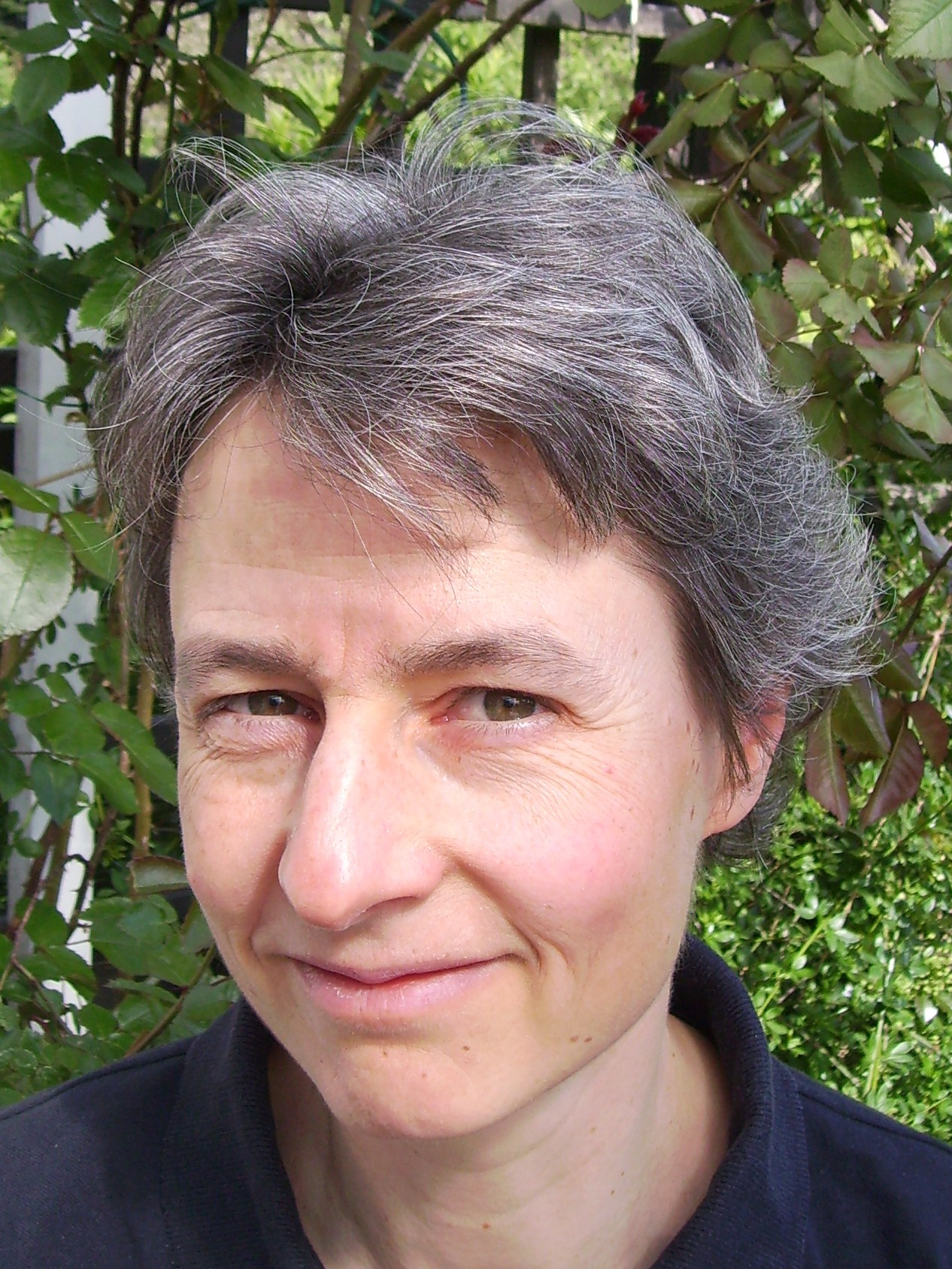I am Professor at the
Lehrstuhl D für Mathematik of the RWTH Aachen.
My current research interests are
integral representations of finite groups,
lattices, spherical designs and modular forms,
and their analogues in coding theory.
You will find a brief CV (in german) if you click here.
Current editorships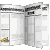Publications
Students
Lectures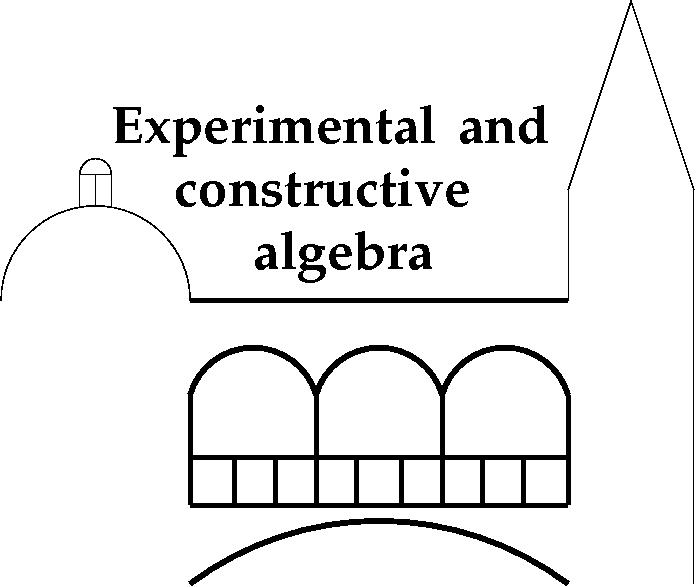The research training group Experimental and constructive algebra started in october 2010.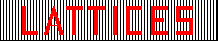Together with Neil J. A. Sloane we are building a database of interesting lattices

On august 11 2010 I found an extremal even unimodular lattice of dimension 72. A new version of the paper can be downloaded here.
Magma code to construct this lattice is found here
Construction of the extremal lattice.
Computation of super offenders in all 9 lattices
Computation of the norm 6 vectors in all 9 lattices using an orthogonally decomposable overlattice.

In the paper On Siegel modular forms of weight 12 ( pdf-file) with B. Venkov,
we calculate the action of some Hecke operators on the space generated by the 24 Niemeier lattices.
You get the Hecke operators and the eigenforms in maple format if you click here.
The structure constants for the multiplication of the eigenforms are in the big maple-file multiplication.
The absolutely irreducible maximal finite subgroups of GL(n,D)
for definite quaternion algebras D of dimension less than 40/n over the rationals
are classified in my paper Finite quaternionic matrix groups. ( pdf-file of a preprint)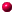linksMAGMAATLASThe first colombian workshop in coding theoryThe number theory webWomen in Number TheoryLANL Preprint ArchiveBiographies of women mathematiciansArchiv der MathematikDiese Seite ist auch auf Deutsch erhältlich.